# 1. Let X and Y be two jointly continuous random variables with joint CDF otherwsie a....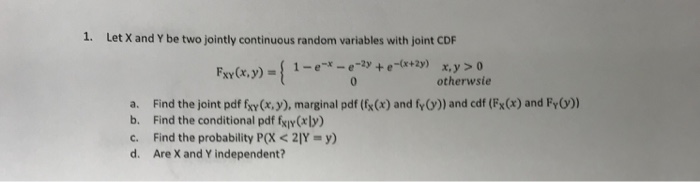1. Let X and Y be two jointly continuous random variables with joint CDF otherwsie a. Find the joint pdf fxy(x, y), marginal pdf (fx(x) and fy()) and cdf (Fx(x) and Fy)) b. Find the conditional pdf fxiy Cr ly c. Find the probability P(X < Y = y) d. Are X and Y independent?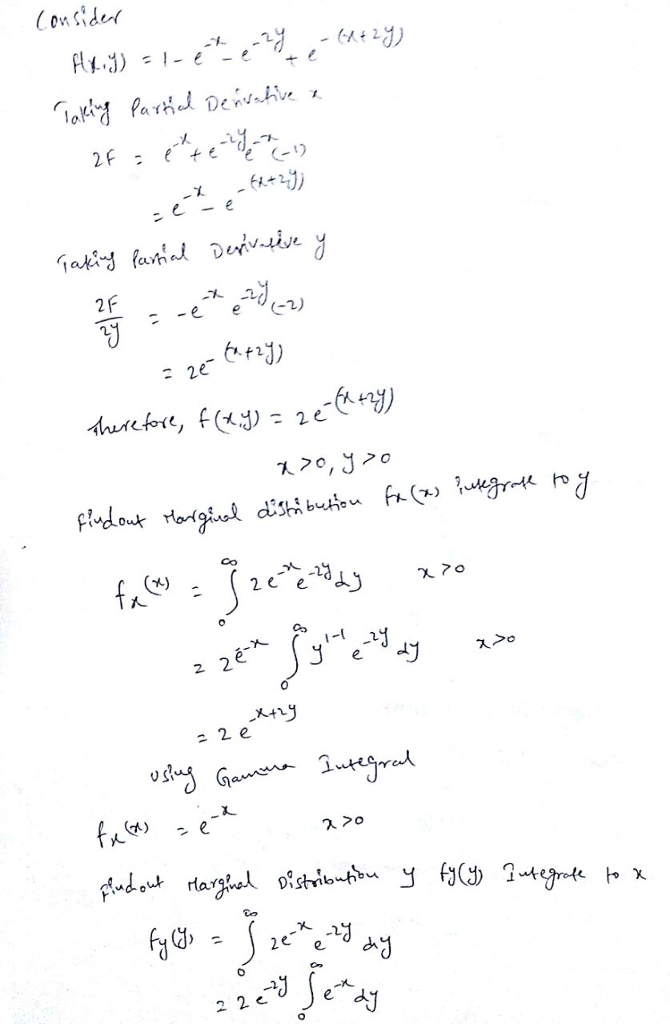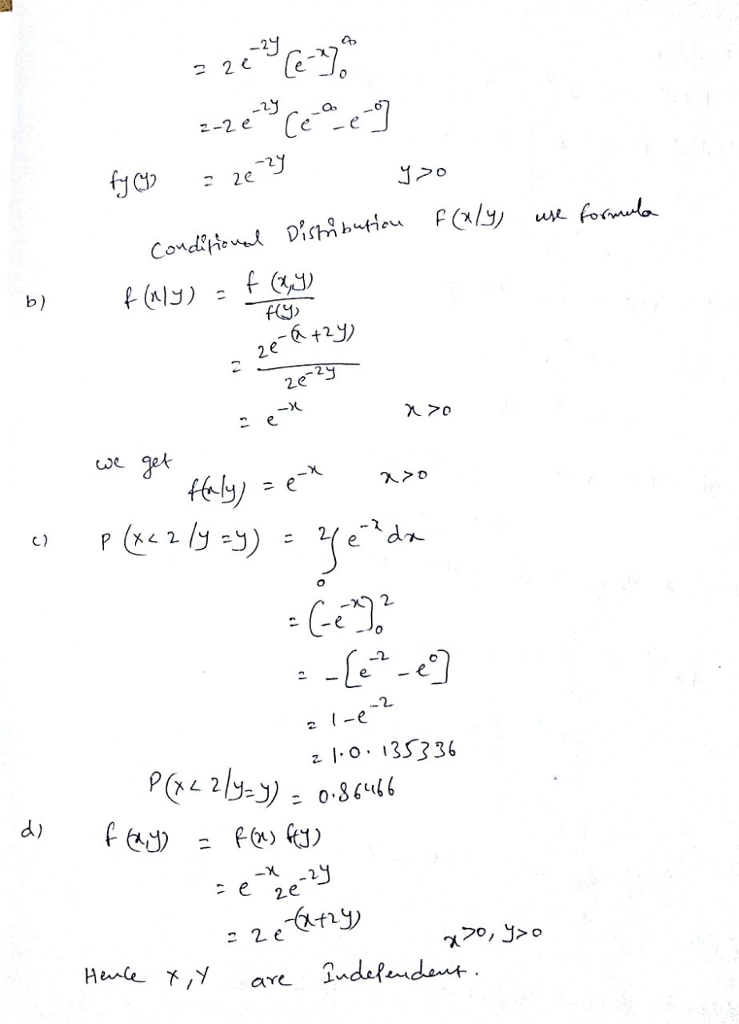##### Add Answer to: 1. Let X and Y be two jointly continuous random variables with joint CDF otherwsie a....
Similar Homework Help Questions
• ### Let X and Y be two jointly continuous random variables with joint PDF xy0x, y <...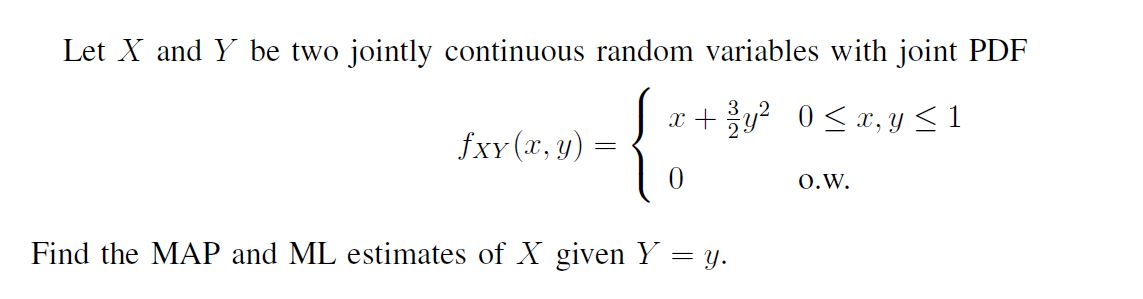Let X and Y be two jointly continuous random variables with joint PDF xy0x, y < 1 fxy (x, y) O.W Find the MAP and ML estimates of X given Y = y

• ### 55. Let X and Y be jointly continuous random variables with joint density function fx.y(x,y) be-3y...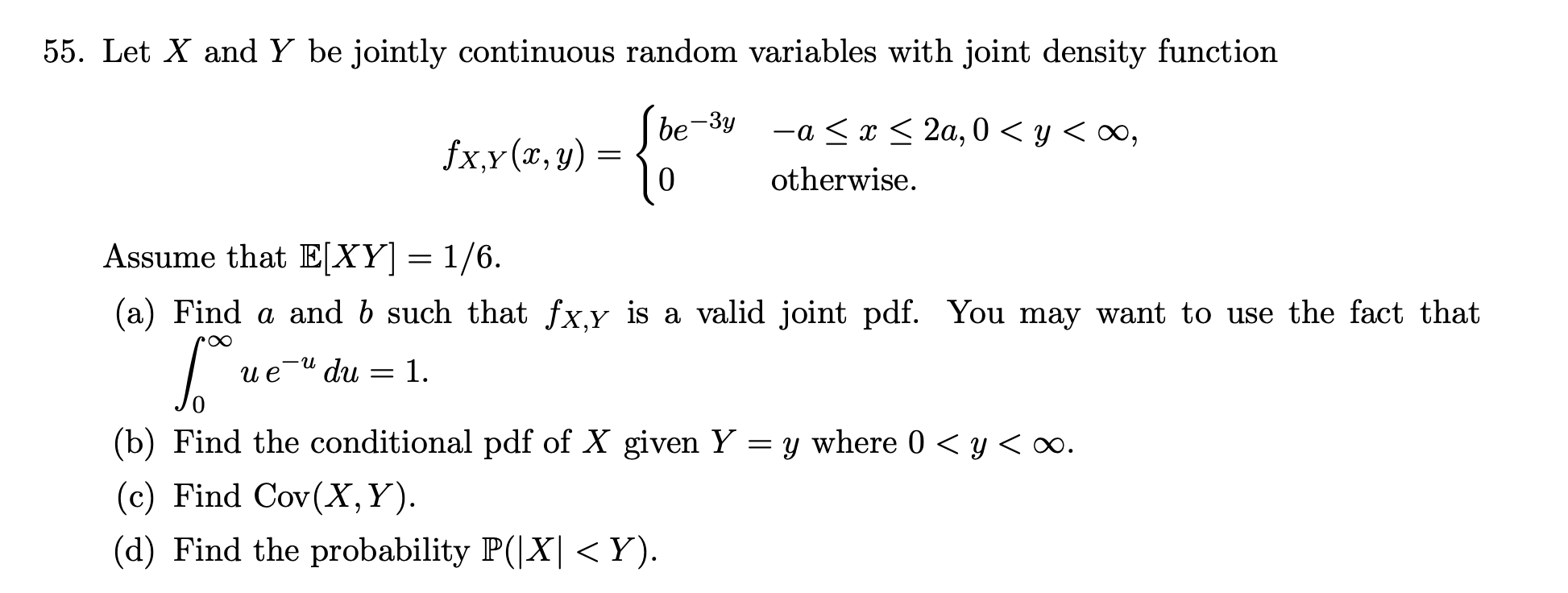55. Let X and Y be jointly continuous random variables with joint density function fx.y(x,y) be-3y -a < x < 2a, 0) < y < 00, otherwise. Assume that E[XY] = 1/6. (a) Find a and b such that fx,y is a valid joint pdf. You may want to use the fact that du = 1. u 6. и е (b) Find the conditional pdf of X given Y = y where 0 <y < . (c) Find Cov(X,Y). (d)...

• ### Let X, Y be jointly continuous with joint density function (pdf) fx,y(x, y) *(1+xy) 05 x...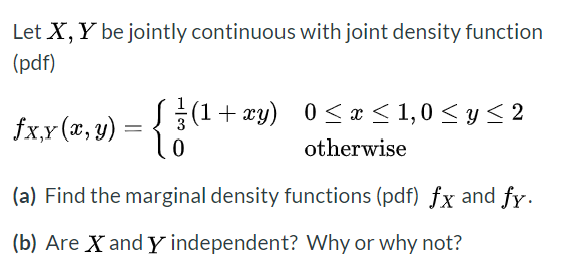Let X, Y be jointly continuous with joint density function (pdf) fx,y(x, y) *(1+xy) 05 x <1,0 <2 0 otherwise (a) Find the marginal density functions (pdf) fx and fy. (b) Are X and Y independent? Why or why not?

• ### Let X and Y be continuous random variables with joint pdf f(x,y) =fX (c(X + Y),...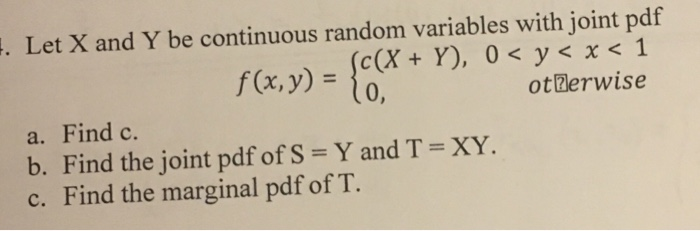Let X and Y be continuous random variables with joint pdf f(x,y) =fX (c(X + Y), 0 < y < x <1 otBerwise a. Find c. b. Find the joint pdf of S = Y and T = XY. c. Find the marginal pdf of T. 、

• ### 2. Let the random variables X and Y have the joint PDF given below: 2e -y...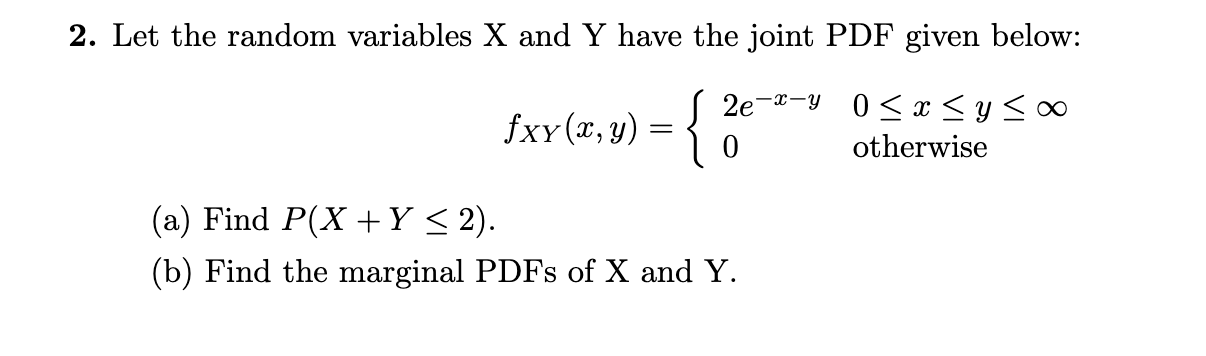2. Let the random variables X and Y have the joint PDF given below: 2e -y 0 xyo0 fxy (x, y) otherwise 0 (a) Find P(X Y < 2) (b) Find the marginal PDFs of X and Y (c) Find the conditional PDF of Y X x (d) Find P(Y< 3|X = 1)

• ### 2. Let X and Y be two continuous random variables varying in accordance with the joint...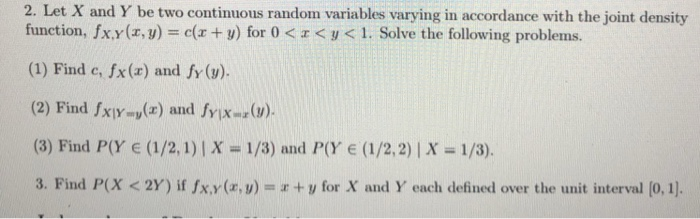2. Let X and Y be two continuous random variables varying in accordance with the joint density function, fx.y(z, y-e(x + y) for 0 < z < y < 1. Solve the following problem s. (1) Find e, fx(a) and fy (v) (2) Find fx-u(z) and fY1Xux(y) (8) Find P(Y e (1/2, 1)|X -1/3) and P(Y e (1/2,2)| X 1/3). 3. Find P(X < 2Y) if fx.y(zw) = x + U for X and Y each defined over the unit...

• ###  The joint probability density function of two continuous random variables X and Y is fxy(x,...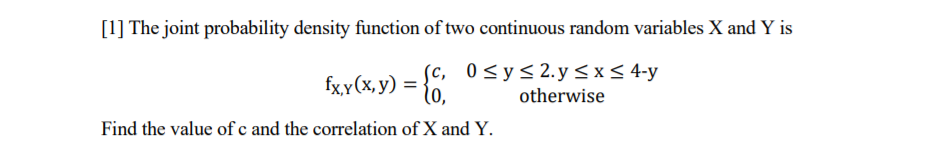The joint probability density function of two continuous random variables X and Y is fxy(x, y) = {0. sc, 0 <y s 2.y < x < 4-y = otherwise Find the value of c and the correlation of X and Y.

• ### 1. (10 pts) Two discrete random variables X and Y are jointly distributed. The joint pmf...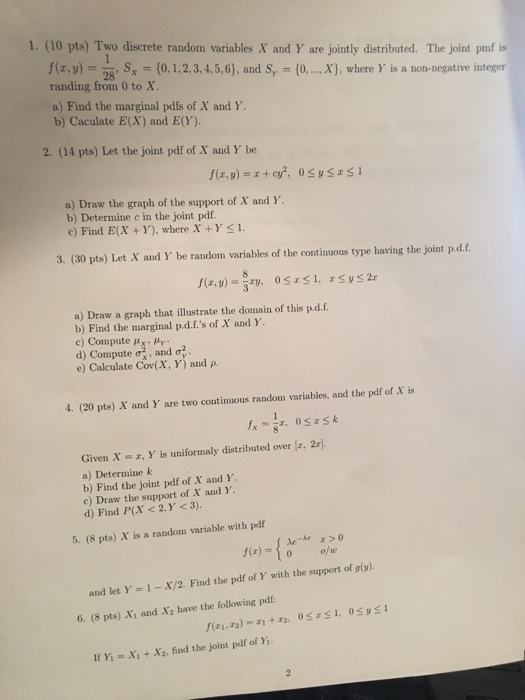1. (10 pts) Two discrete random variables X and Y are jointly distributed. The joint pmf is f(z, y) = 2 , Sx = {0. 1. 2. 3. 4.5.6), and Sr = {0, randing from 0 to X. X), where Y is a non-negative integer a) Find the marginal pdfs of X and Y b) Caculate E(X) and E(Y). 2. (14 pts) Let the joint pdf of X aud Y be a) Draw the graph of the support of X...

• ### 2. Let the random variables X and Y have the joint PDF given below: S 2e-2-Y...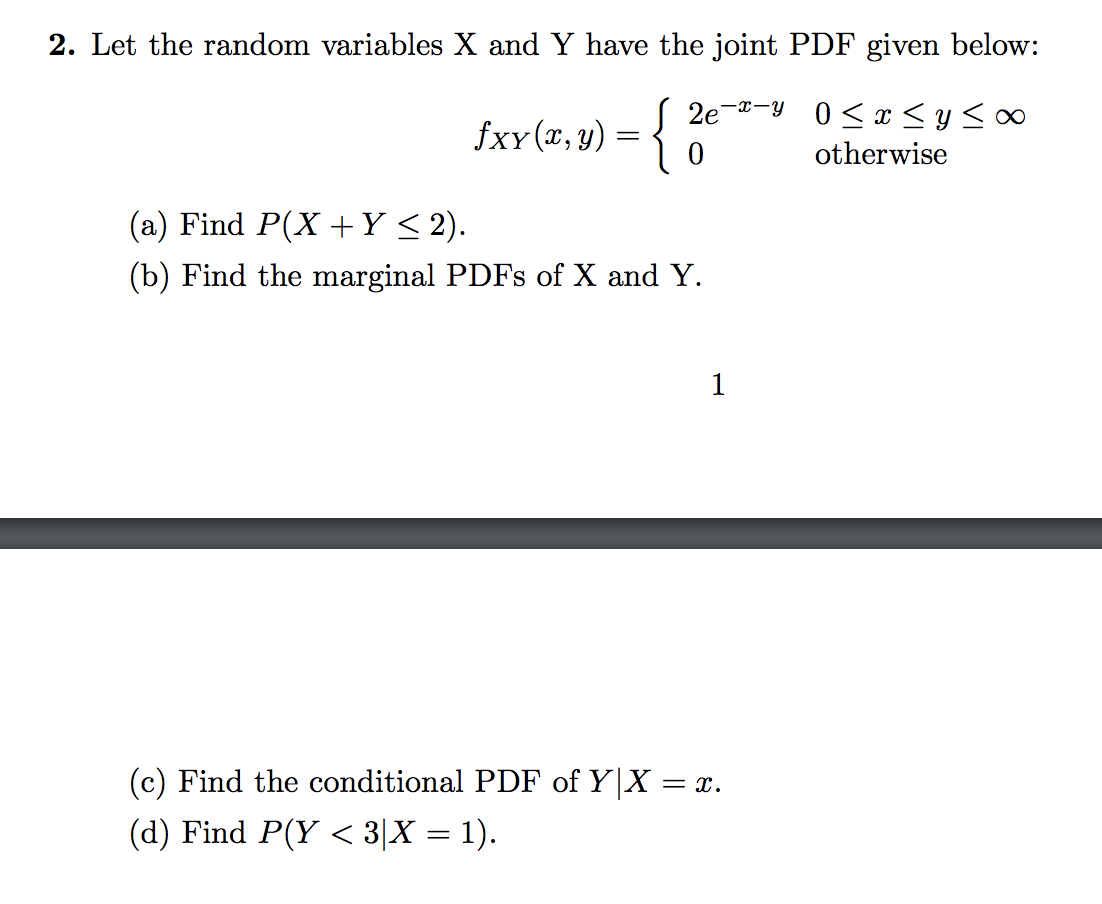2. Let the random variables X and Y have the joint PDF given below: S 2e-2-Y 0 < x < y < fxy(x,y) = { 0 otherwise (a) Find P(X+Y < 2). (b) Find the marginal PDFs of X and Y. (c) Find the conditional PDF of Y|X = r. (d) Find P(Y <3|X = 1).

• ### The joint probability density function of two continuous random variables X and Y is Find the...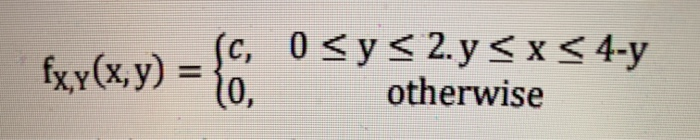The joint probability density function of two continuous random variables X and Y is Find the value of c and the correlation of X and Y. Consider the same two random variables X and Y in problem  with the same joint probability density function. Find the mean value of Y when X<1. fxy(x,y) = { C, 0 <y < 2.y < x < 4-y 10, otherwise

Free Homework App